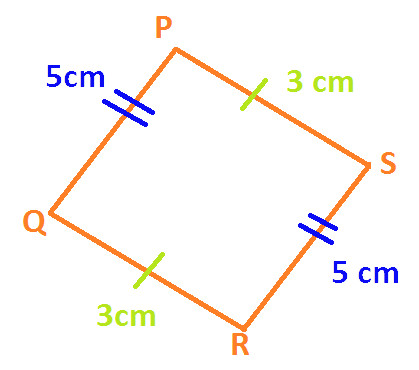Home >> Parallelogram >>

Define Parallelogram

Area of Parallelogram Opposite Angles of Parallelogram Adjacent Angles of Parallelogram Diagonal Of Parallelogram Difference & Similarity between Rectangle & Parallelogram Difference & Similarity between Square & Parallelogram Difference & Similarity between Square, Rectangle & Parallelogram Properties of Parallelogram

Before you study what is Parallelogram, you are advised to read:

What is a Quadrilateral ?
What are Parallel Lines ?

Definition of Parallelogram : A parallelogram is a quadrilateral in which opposite sides are equal and parallel to each other.

Example 1: In the following diagram of Quadrilateral ABCDAB is parallel to DC
AD is parallel to BC
AB = DC = 7 cm
AD = BC = 11 cm

Hence, we can say Quadrilateral ABCD is a parallelogram.

Example 2: In the following diagram of Quadrilateral PQRSPQ is parallel to SR
PS is parallel to QR

PQ = SR = 5 cm
PS = QR = 3 cm

Hence, we can say Quadrilateral PQRS is a parallelogram.